Ex 13.5 (Optional)

Chapter 13 Class 10 Surface Areas and Volumes
Serial order wise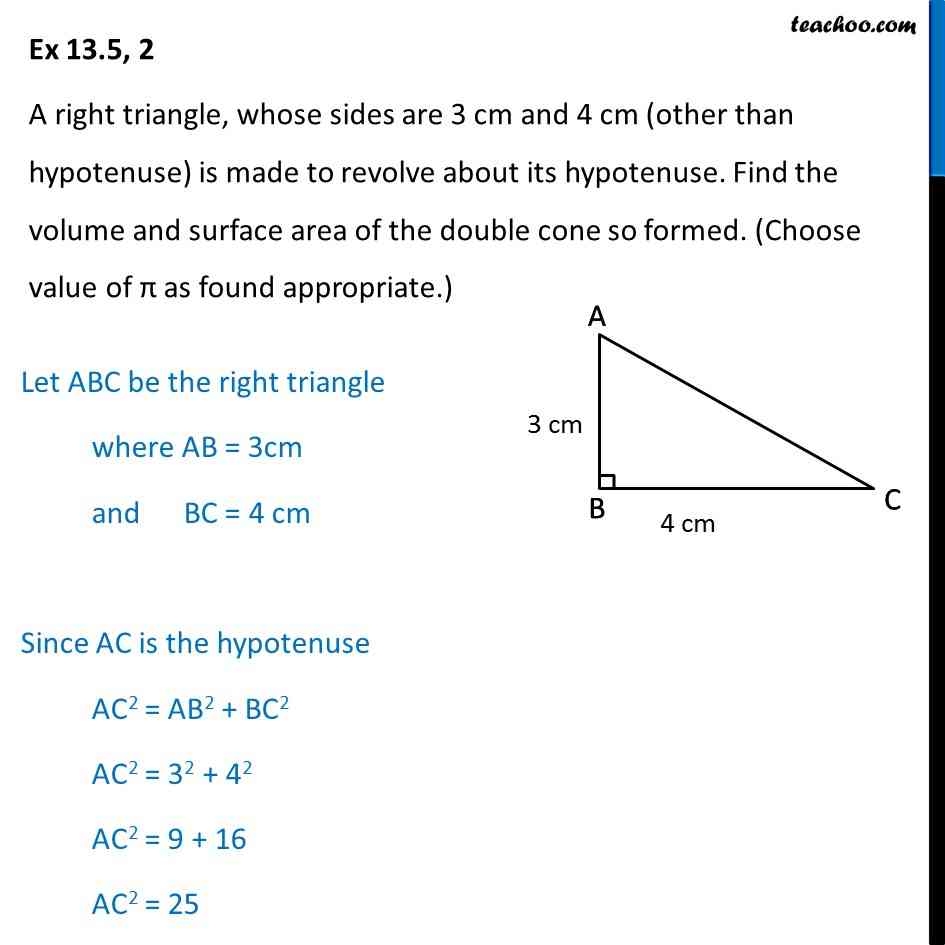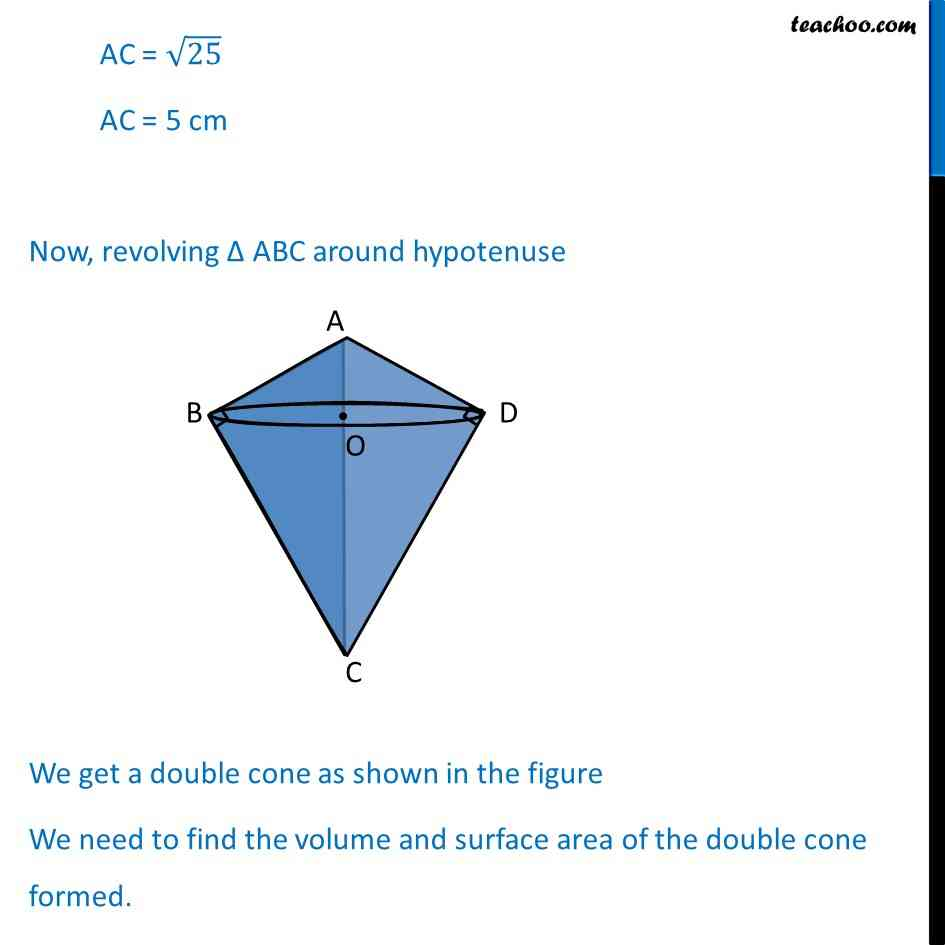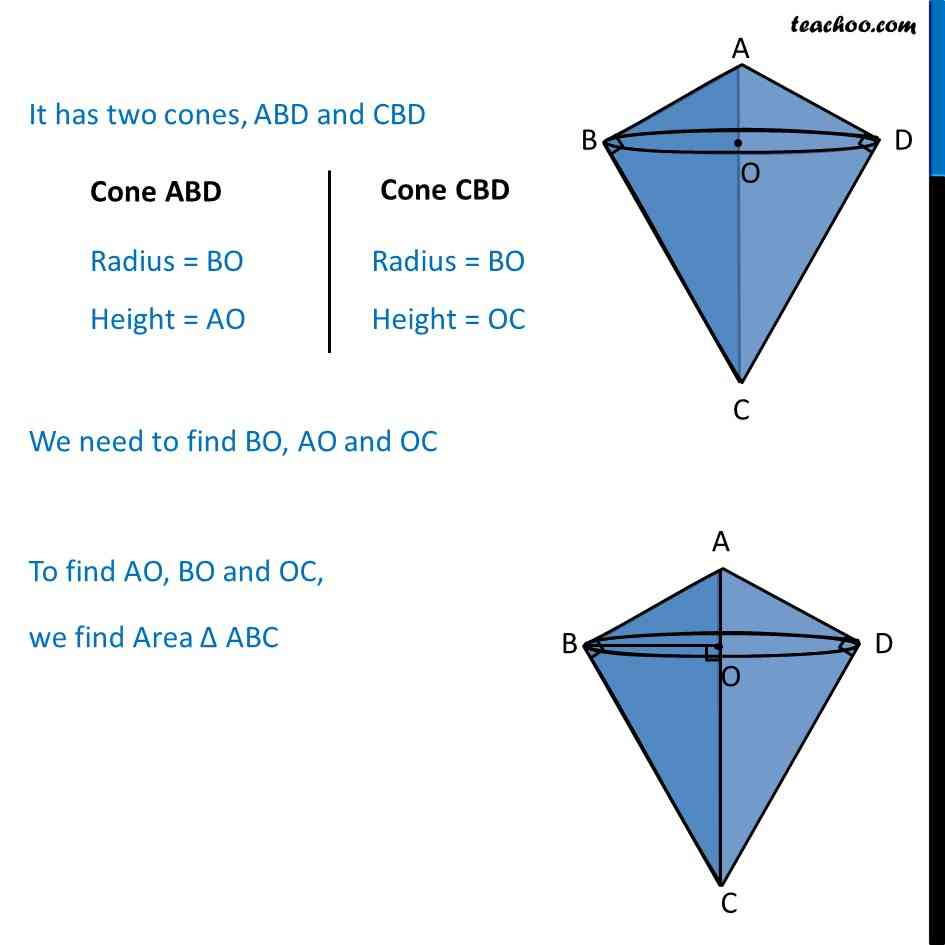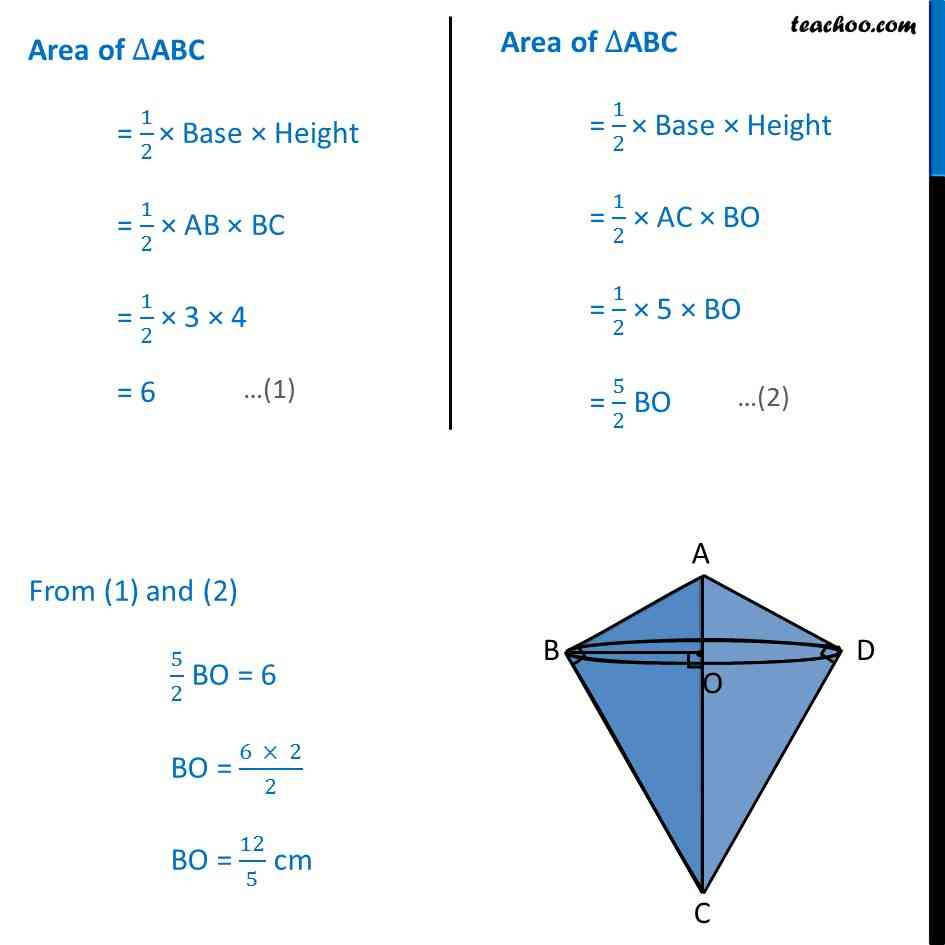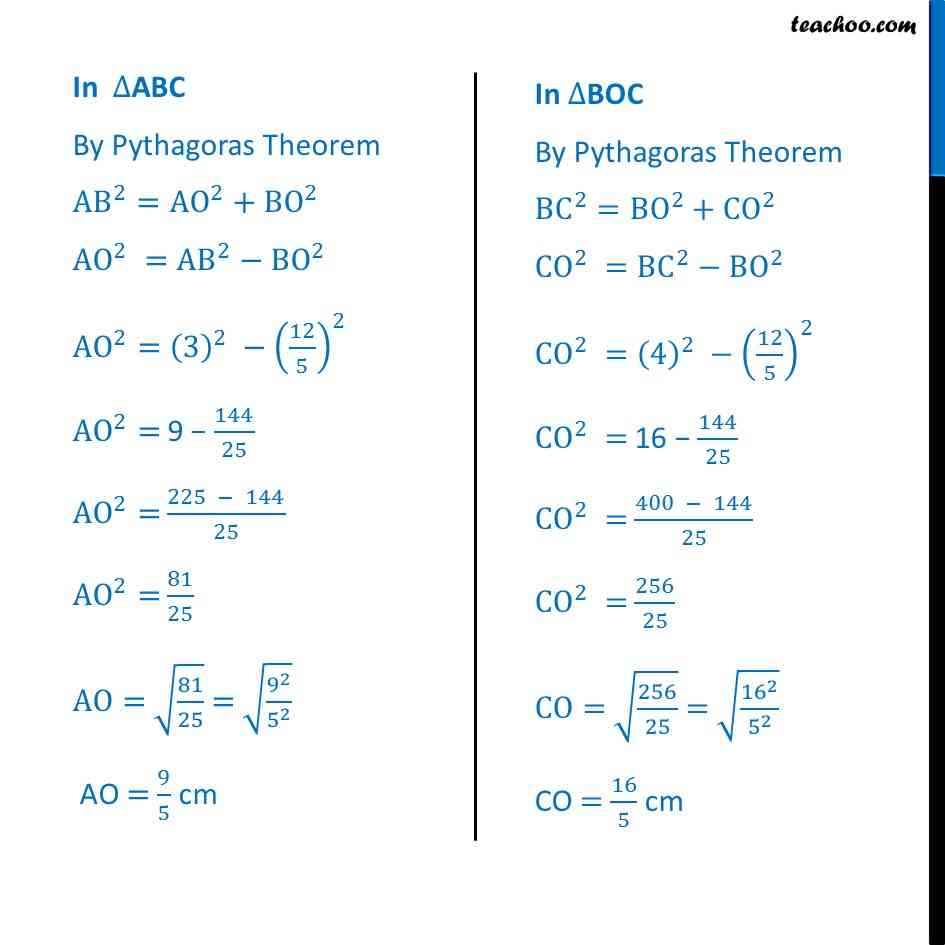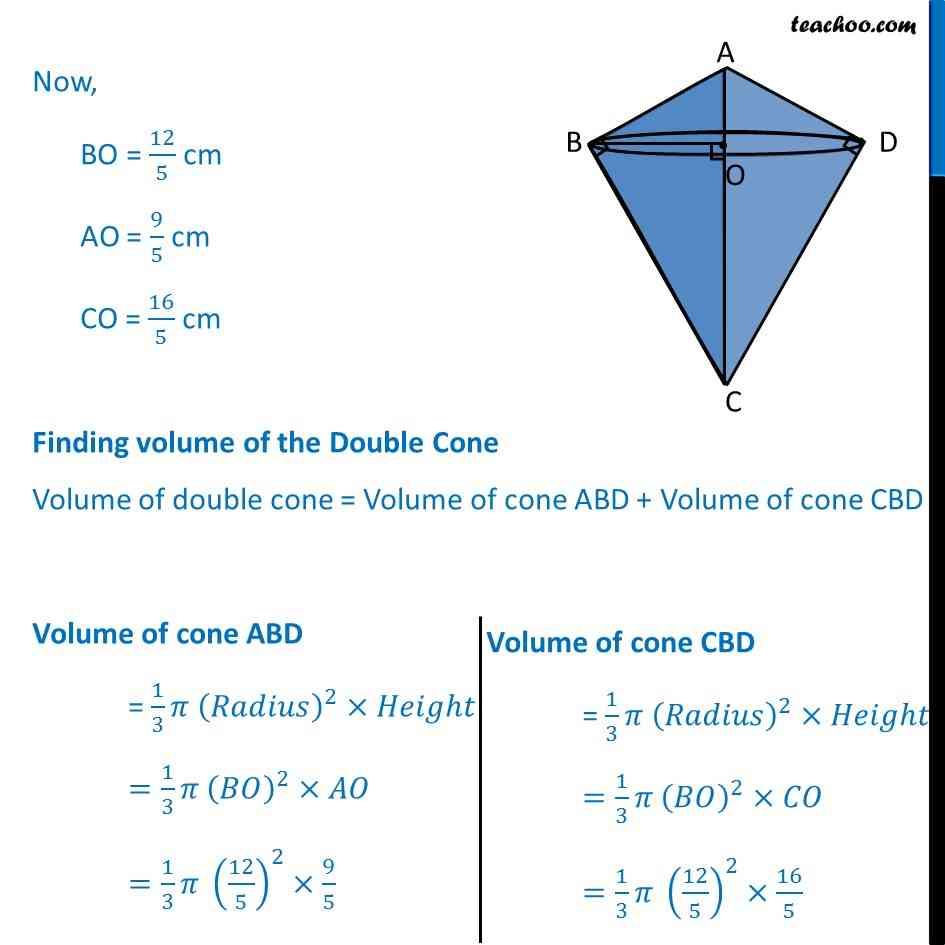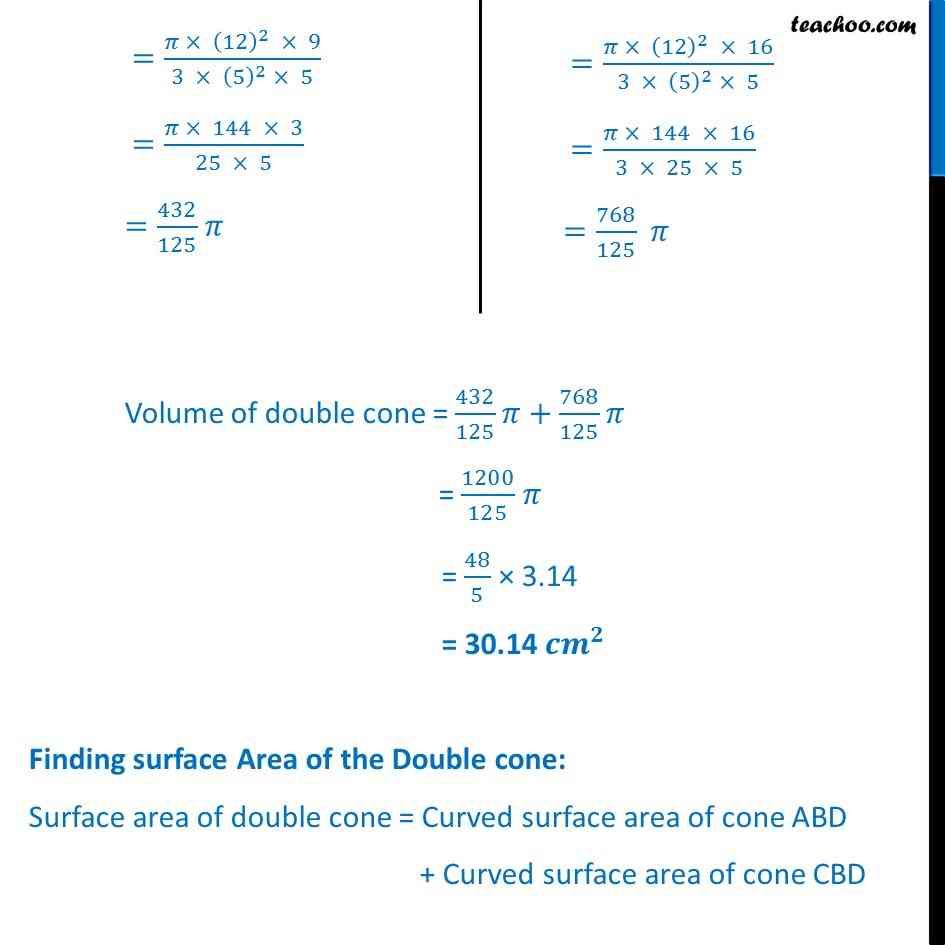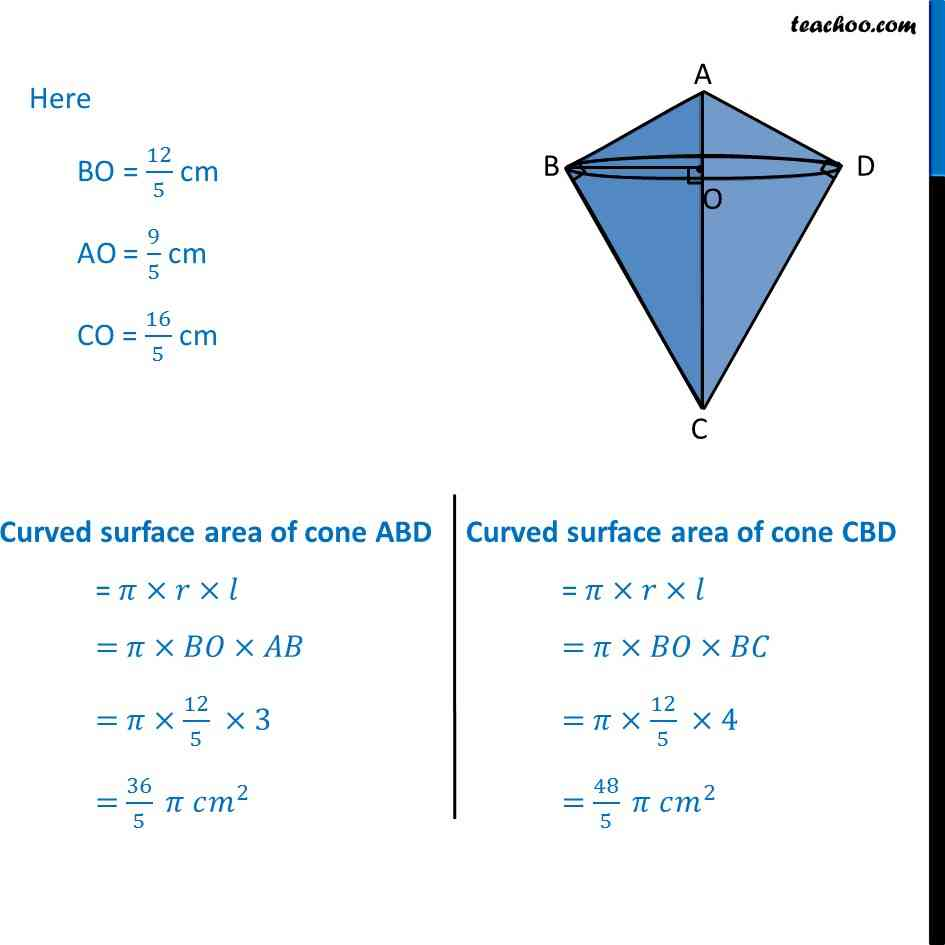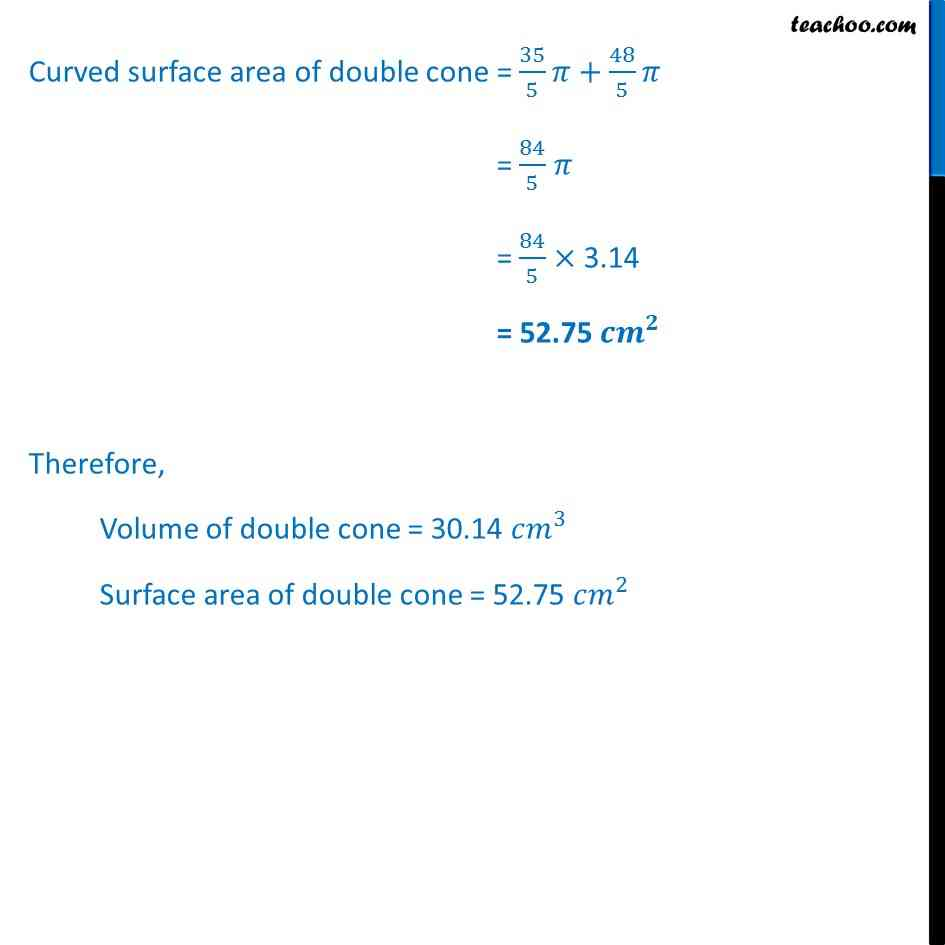Get live Maths 1-on-1 Classs - Class 6 to 12

### Transcript

Ex 13.5, 2 A right triangle, whose sides are 3 cm and 4 cm (other than hypotenuse) is made to revolve about its hypotenuse. Find the volume and surface area of the double cone so formed. (Choose value of as found appropriate.) Let ABC be the right triangle where AB = 3cm and BC = 4 cm Since AC is the hypotenuse AC2 = AB2 + BC2 AC2 = 32 + 42 AC2 = 9 + 16 AC2 = 25 AC = 25 AC = 5 cm Now, revolving ABC around hypotenuse We get a double cone as shown in the figure We need to find the volume and surface area of the double cone formed. It has two cones, ABD and CBD We need to find BO, AO and OC To find AO, BO and OC, we find Area ABC Cone ABD Radius = BO Height = AO Cone CBD Radius = BO Height = OC Area of ABC = 1/2 " " Base Height = 1/2 AB BC = 1/2 3 4 = 6 Area of ABC = 1/2 " " Base Height = 1/2 AC BO = 1/2 5 BO = 5/2 BO From (1) and (2) 5/2 BO = 6 BO = (6 2)/2 BO = 12/5 cm In ABC By Pythagoras Theorem AB^2=AO^2+BO^2 AO^2 =AB^2 BO^2 AO^2=(3)^2 (12/5)^2 AO^2= 9 144/25 AO^2= (225 144)/25 AO^2= 81/25 AO= (81/25) = (9^2/5^2 ) AO = 9/5 cm In BOC By Pythagoras Theorem BC^2=BO^2+CO^2 CO^2 =BC^2 BO^2 CO^2 =(4)^2 (12/5)^2 CO^2 = 16 144/25 CO^2 = (400 144)/25 CO^2 = 256/25 CO= (256/25) = ( 16 ^2/5^2 ) CO = 16/5 cm Now, BO = 12/5 cm AO = 9/5 cm CO = 16/5 cm Finding volume of the Double Cone Volume of double cone = Volume of cone ABD + Volume of cone CBD Volume of cone ABD = 1/3 ( )^2 =1/3 ( )^2 =1/3 (12/5)^2 9/5 =( (12)^2 9)/(3 (5)^2 5) =( 144 3)/(25 5) =432/125 Volume of cone CBD = 1/3 ( )^2 =1/3 ( )^2 =1/3 (12/5)^2 16/5 =( (12)^2 16)/(3 (5)^2 5) =( 144 16)/(3 25 5) =768/125 Volume of double cone = 432/125 +768/125 = 1200/125 = 48/5 3.14 = 30.14 ^ Finding surface Area of the Double cone: Surface area of double cone = Curved surface area of cone ABD + Curved surface area of cone CBD Here BO = 12/5 cm AO = 9/5 cm CO = 16/5 cm Curved surface area of cone ABD = = = 12/5 3 = 36/5 ^2 Curved surface area of cone CBD = = = 12/5 4 = 48/5 ^2 Curved surface area of double cone = 35/5 +48/5 = 84/5 = 84/5 3.14 = 52.75 ^ Therefore, Volume of double cone = 30.14 ^3 Surface area of double cone = 52.75 ^2